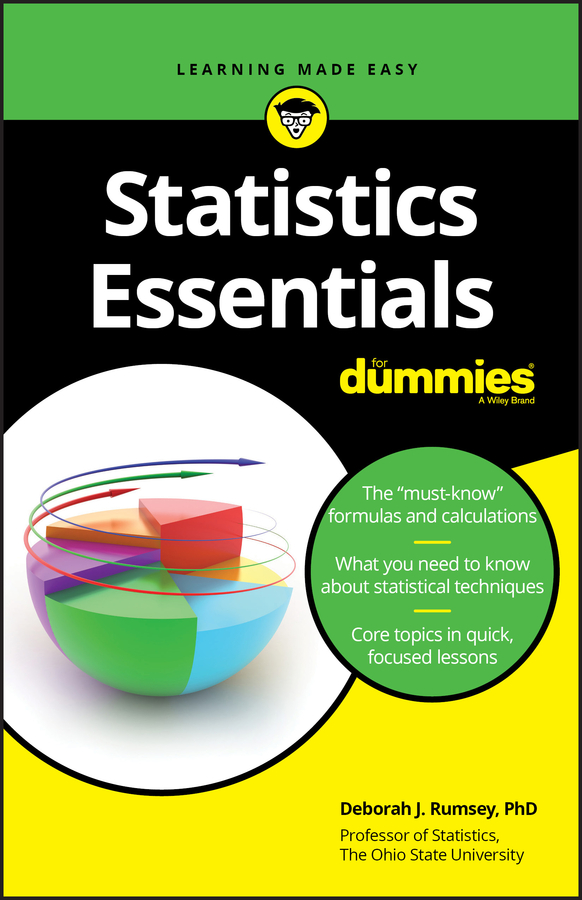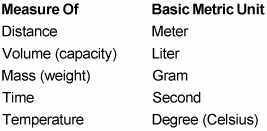##### Statistics Essentials For DummiesThe metric system provides units of measurement for distance, volume, mass, time, and temperature. It builds these units using a basic unit and a set of prefixes.

The following list shows five important basic units in the metric system.The following table shows ten metric prefixes, with the three most commonly used in bold.

Prefix Meaning Number Power of Ten
Giga- One billion 1,000,000,000 109
Mega- One million 1,000,000 106
Kilo- One thousand 1,000 103
Hecta- One hundred 100 102
Deca- Ten 10 101
(none) One 1 100
Deci- One tenth 0.1 10-1
Centi- One hundredth 0.01 10-2
Milli- One thousandth 0.001 10-3
Micro- One millionth 0.000001 10-6
Nano- One billionth 0.000000001 10-9
Large and small metric units are formed by linking a basic unit with a prefix. For example, linking the prefix kilo- to the basic unit meter gives you the kilometer, which means 1,000 meters. Similarly, linking the prefix milli- to the basic unit liter gives you the milliliter, which means 0.001 (one thousandth) of a liter.

Here’s a list giving you the basics:

• Units of distance: The basic metric unit of distance is the meter (m). Other common units are millimeters (mm), centimeters (cm), and kilometers (km):

1 kilometer = 1,000 meters

1 meter = 100 centimeters

1 meter = 1,000 millimeters

• Units of fluid volume: The basic metric unit of fluid volume (also called capacity) is the liter (L). Another common unit is the milliliter (mL):

1 liter = 1,000 milliliters

Note: One milliliter is equal to 1 cubic centimeter (cc).

• Units of mass: Technically speaking, the metric system measures not weight but mass. Weight is the measurement of how strongly gravity pulls an object toward Earth. Mass, however, is the measurement of the amount of matter an object has. If you traveled to the moon, your weight would change, so you would feel lighter. But your mass would remain the same, so all of you would still be there. Unless you’re planning a trip into outer space or performing a scientific experiment, you probably don’t need to know the difference between weight and mass.

The basic unit of weight in the metric system is the gram (g). Even more commonly used, however, is the kilogram (kg):

1 kilogram = 1,000 grams

Note: 1 kilogram of water has a volume of 1 liter.

• Units of time: As in the English system, the basic metric unit of time is a second (s). For most purposes, people also use other English units such as minutes, hours, and so forth.

For many scientific purposes, the second is the only unit used to measure time. Large numbers of seconds and small fractions of sections are represented with scientific notation.

• Units of speed: For most purposes, the most common metric unit of speed (also called velocity) is kilometers per hour (km/hr). Another common unit is meters per second (m/s).

• Units of temperature (degrees Celsius or Centigrade): The basic metric unit of temperature is the Celsius degree (°C), also called the Centigrade degree. The Celsius scale is set up so that at sea level, water freezes at 0°C and boils at 100°C.

Scientists often use another unit — the Kelvin (K) — to talk about temperature. The degrees are the same size as in Celsius, but 0 K is set at absolute zero, the temperature at which atoms don’t move at all. Absolute zero is approximately equal to –273.15°C.